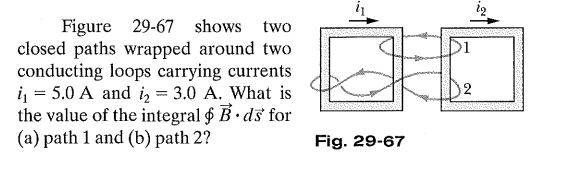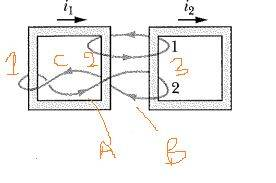# Ampere's law -- application with two conducting loops

Physicslearner500039
Homework Statement:
Figure 29-67 shows two closed paths wrapped around two conducting loops carrying currents
i j = 5.0 A and i2 = 3.0 A. What is the value of the integral ~ B· d'S for (a) path 1 and (b) path2?
Relevant Equations:
No specific equations
I am trying to get more confidence on the direction of current using Amperes law, the problem statement isLoop1:
My first task was to assign the direction of current. If I wrap around the my right hand fingers in the direction of integration the thumb is pointing up hence Positive Y direction current is +Ve and Negative Y direction current is -Ve. Hence i1 is -ve and i2 is +Ve.
∫B.ds = (-5+3)*μ = -2*4*π*10^-7 = -2.5u T.m.
Is everything ok?

Loop2:
The real confusion happens here while assigning the current direction I have shown the integration pointsRight hand rules for integration points
A: Up current is positive.
B: Up current is negative.
C: Up current is positive.
So which direction should i consider positive?
For side 1 the current is one direction and for side 2 it is opposite direction. Do they always cancel? Please advise.

•